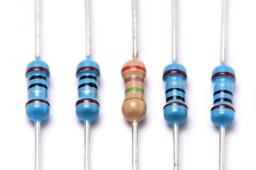# Resistances 80706

The current I = 4A branches into three wires with resistances R1 = 2Ώ, R2 = 5Ώ, R3 = 10Ώ. Calculate the total resistance of the connection and the voltage of the source. How big are the currents in each wire?

R =  1.25 Ω
U =  5 V
I1 =  2.5 A
I2 =  1 A
I3 =  0.5 A

### Step-by-step explanation:Did you find an error or inaccuracy? Feel free to write us. Thank you!

Tips for related online calculators
Check out our ratio calculator.